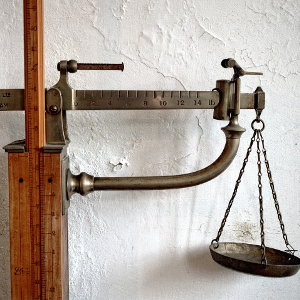# Pounds to Kilograms & Grams Unit Converter / Lb to Kg and G Calculator

Share:

Pounds
lb
Kilograms
kg
Grams
g

Kilograms
kg
Grams
g
Pounds
lb

Result:

Pounds
lb
Kilograms
kg

Kilogram
kg
Pounds
lb

Result:

Pounds
lb
Grams
g

Grams
g
Pounds
lb

Result:

## Pound(lb), Gram(g) & Kilogram(kg) Conversion Chart

### Generating And Printing The Pound(lb) & Kilogram(kg) Conversion Chart

1. Select the Distance/Length conversion you want to perform from the drop down box Conversion Type. You can convert from Pound to Kilogram and vice versa.
2. Select the decimal place rounding in the Rounding drop down. By default, this converter uses 3 decimal places.
3. Enter the Starting Distance you desire to start the conversion chart.
4. Enter the Interval between the distance. Example: -100, -10, 0.25, 0.5, 1, 10, 100, etc...
5. Click the Generate Chart button to generate your desired chart. See list of Pound conversion charts you can generate .
6. Click the Print button to print the chart you have generated. This printing option allows you to print only the chart portion.

Conversion Type
Round To
Digit Separation
Start. Value
lb
Increment
lb

Pound Kilogram
1lb 0kg 453.592g
2lb 0kg 907.185g
3lb 1kg 360.777g
4lb 1kg 814.369g
5lb 2kg 267.962g
6lb 2kg 721.554g
7lb 3kg 175.147g
8lb 3kg 628.739g
9lb 4kg 82.331g
10lb 4kg 535.924g
11lb 4kg 989.516g
12lb 5kg 443.108g
13lb 5kg 896.701g
14lb 6kg 350.293g
15lb 6kg 803.886g
16lb 7kg 257.478g
17lb 7kg 711.07g
18lb 8kg 164.663g
19lb 8kg 618.255g
20lb 9kg 71.847g
21lb 9kg 525.44g
22lb 9kg 979.032g
23lb 10kg 432.625g
24lb 10kg 886.217g
25lb 11kg 339.809g
Pound Kilogram
26lb 11kg 793.402g
27lb 12kg 246.994g
28lb 12kg 700.586g
29lb 13kg 154.179g
30lb 13kg 607.771g
31lb 14kg 61.363g
32lb 14kg 514.956g
33lb 14kg 968.548g
34lb 15kg 422.141g
35lb 15kg 875.733g
36lb 16kg 329.325g
37lb 16kg 782.918g
38lb 17kg 236.51g
39lb 17kg 690.102g
40lb 18kg 143.695g
41lb 18kg 597.287g
42lb 19kg 50.88g
43lb 19kg 504.472g
44lb 19kg 958.064g
45lb 20kg 411.657g
46lb 20kg 865.249g
47lb 21kg 318.841g
48lb 21kg 772.434g
49lb 22kg 226.026g
50lb 22kg 679.618g
Pound Kilogram
51lb 23kg 133.211g
52lb 23kg 586.803g
53lb 24kg 40.396g
54lb 24kg 493.988g
55lb 24kg 947.58g
56lb 25kg 401.173g
57lb 25kg 854.765g
58lb 26kg 308.357g
59lb 26kg 761.95g
60lb 27kg 215.542g
61lb 27kg 669.135g
62lb 28kg 122.727g
63lb 28kg 576.319g
64lb 29kg 29.912g
65lb 29kg 483.504g
66lb 29kg 937.096g
67lb 30kg 390.689g
68lb 30kg 844.281g
69lb 31kg 297.874g
70lb 31kg 751.466g
71lb 32kg 205.058g
72lb 32kg 658.651g
73lb 33kg 112.243g
74lb 33kg 565.835g
75lb 34kg 19.428g
Pound Kilogram
76lb 34kg 473.02g
77lb 34kg 926.612g
78lb 35kg 380.205g
79lb 35kg 833.797g
80lb 36kg 287.39g
81lb 36kg 740.982g
82lb 37kg 194.574g
83lb 37kg 648.167g
84lb 38kg 101.759g
85lb 38kg 555.351g
86lb 39kg 8.944g
87lb 39kg 462.536g
88lb 39kg 916.129g
89lb 40kg 369.721g
90lb 40kg 823.313g
91lb 41kg 276.906g
92lb 41kg 730.498g
93lb 42kg 184.09g
94lb 42kg 637.683g
95lb 43kg 91.275g
96lb 43kg 544.868g
97lb 43kg 998.46g
98lb 44kg 452.052g
99lb 44kg 905.645g
100lb 45kg 359.237g

## Types Of Pounds, Kilograms & Grams Conversion ChartsBelow are the types of lb, kg & g conversion charts or tables you can generate. You can choose these charts by changing the options in Conversion Type drop down field above.

• Pound (lb) to Kilogram (kg) & Gram (g) conversion chart.
• Pound (lb) to Kilogram (kg) conversion chart.
• Pound (lb) to Gram (g) conversion chart.
• Kilogram (kg) to Pound (lb) conversion chart.
• Gram (g) to Pound (lb) conversion chart.

## Formula For Converting Pound to Kilogram and Gram

### Pound to Gram Conversion Formula

• 1 lb = 453.59237 g (According to the agreement between United Kingdom, U.S.A., and other countries on 1959).

So, if M(g) represents mass in grams and M(lb) represents mass in pounds or lbs, then:

M(g) = 453.59237 × M(lb)

#### Example

For converting 5 lbs to grams:
M(lb) = 5 lbs

M(g) = 453.59237 × M(lb) = 453.59237 × 5 = 2267.96185 g

### Pound to Kilogram Conversion Formula

• 1 lb = 453.59237 g exact.
• 1 kg = 1000 g.

So, if M(g) represents mass in grams, M(kg) represents mass in kilograms and M(lb) represents mass in pounds or lbs, then:

M(g) = 453.59237 × M(lb)

M(kg) = M(g) ÷ 1000

M(kg) = (453.59237 ÷ 1000) × M(lb) = 0.45359237 × M(lb)

M(kg) = 0.45359237 × M(lb)

#### Example

For converting 5 lbs to kg:
M(lb) = 5 lbs

M(kg) = 0.45359237 × M(lb) = 0.45359237 × 5 = 2.26796185 kgs

### Pound to Kilogram and Gram Conversion

• M(kg) = 0.45359237 × M(lb).
• M(kg) = M(g) ÷ 1000.

#### Example

For converting 50 lbs to kgs and grams:
M(lb) = 5 lbs

• M(kg) = 0.45359237 × M(lb) = 0.45359237 × 50 = 22.67961850 kgs.
• 22.67961850 kgs = 22 kgs + 0.67961850 kg = 22 kgs & (0.67961850 × 1000) g = 22 kgs & 679.61850 g.
• So, 50 lbs = 22 kgs & 679.61850 g.